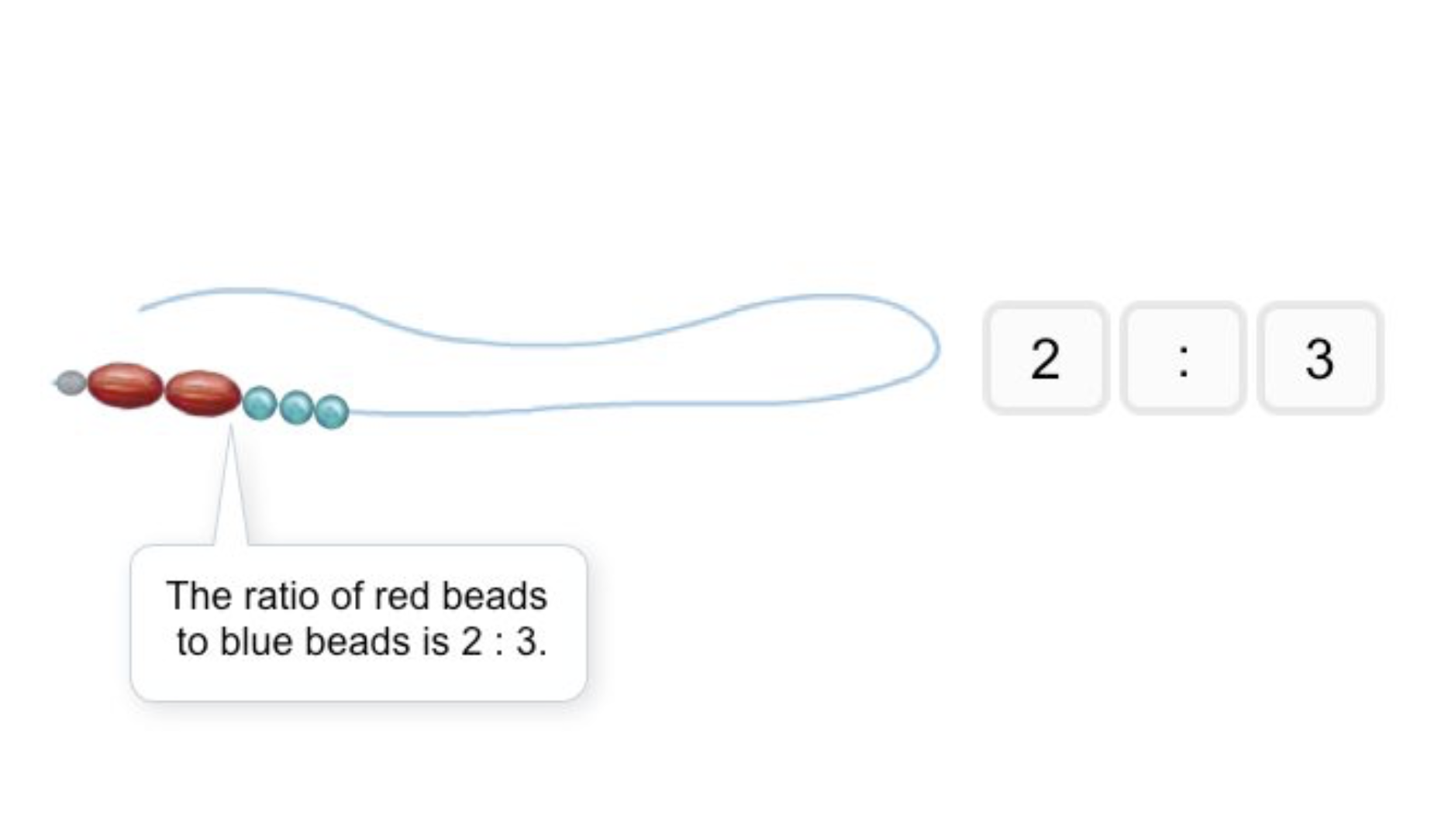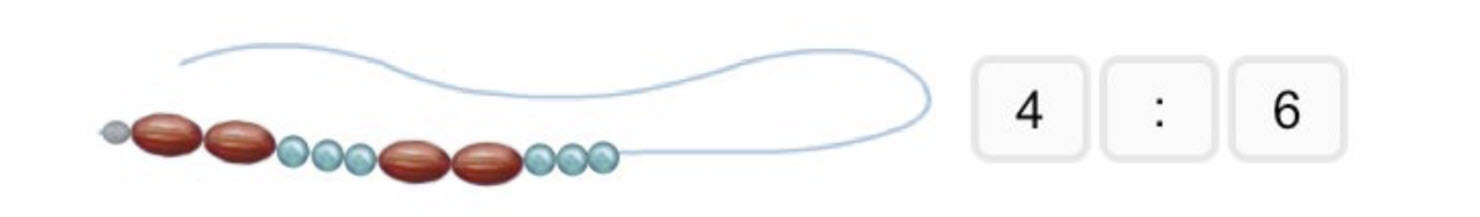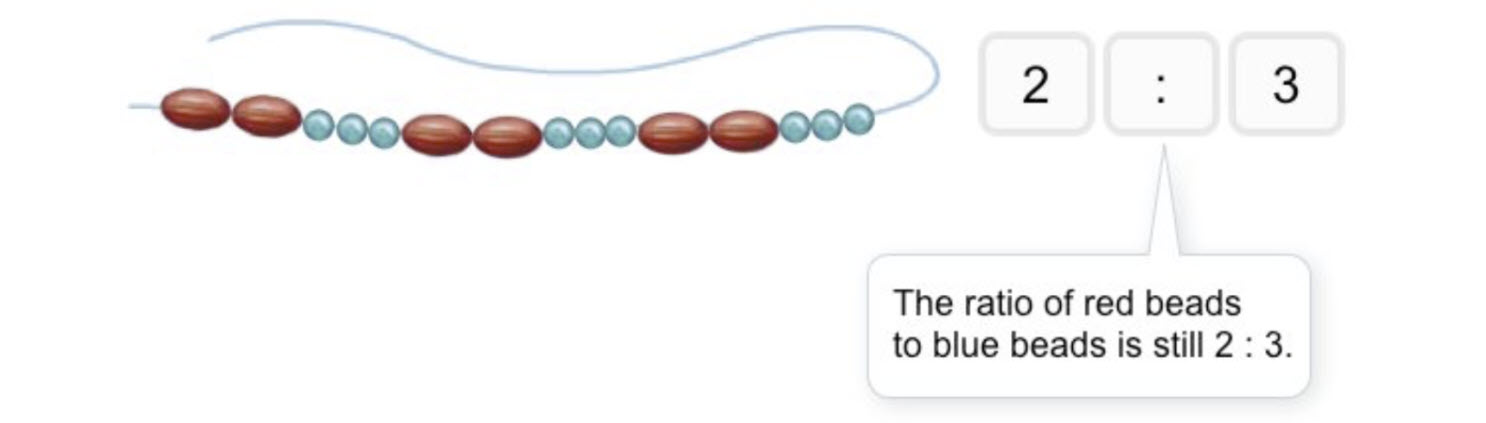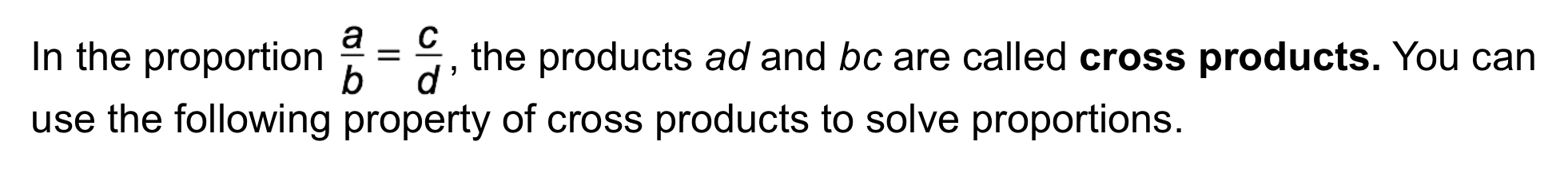Algebra 1 2-7 Guided Practice: Solving Proportions
starstarstarstarstarstarstarstarstarstar
by Matthew Richardson
| 17 Questions1
2
1
10
Solve It! You are making beaded necklaces, using a pattern in which 2 large red beads are followed by 3 small blue beads. Each set of 5 beads adds 1.25 in. to the length of the necklace. How many of each color bead will you need for a 20-in necklace?
48 red & 64 blue
12 red & 16 blue
32 red & 48 blue
16 red & 24 blue
2
103
4
5
6
3
10
Take Note: Define ratio.
4
5
Take Note: Provide an example of a simplified ratio.
5
10
Take Note: Define proportion.
6
5
Take Note: Provide an example of a proportion.
7
10
Problem 1 Got It? What is the solution of the proportion?

6.5
8.75
7.14
5.68
9
10
11
12
8
10
Take Note: Define cross products.
9
5
Take Note: Fill in the blank:

The cross products of a __________ are equal.
fraction
ratio
proportion
10
5
Take Note: Fill in the blank:

Assume that \frac{a}{b}=\frac{c}{d}, b\neq 0, and d\neq0.

Select all that apply.
bc
cb
b+c
11
5
Take Note: Identify the extremes of the proportion. Select all that apply.

g
y
f
x
12
5
Take Note: Identify the means of the proportion. Select all that apply.

g
y
f
x
13
10
Problem 2 Got It? What is the solution of the proportion?

3/15
5/9
5
9/5
14
10
Problem 2 Got It? Reasoning: Would you rather use the Cross Products Property or the Multiplication Property of Equality (aka multiply the numerator and denominator of at least 1 ratio by the same number) to solve the proportion? Explain.

15
10
Problem 3 Got It? What is the solution of the proportion?

-5
-1
1
516
10
Problem 4 Got It? An 8-oz can of orange juice contains about 97 mg of vitamin C.

About how many milligrams of vitamin C are there in a 12-oz can of orange juice?
0.99 mg
64.67 mg
145.5 mg
10.63 mg17
17
10
Take Note: Summarize the mathematical content of this lesson. What topics, ideas, and vocabulary were introduced?